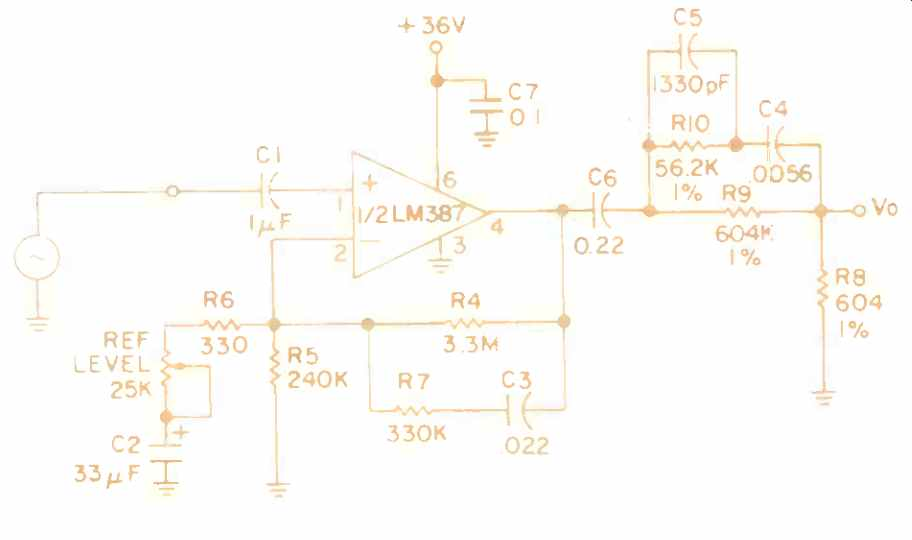# Cheap and Dirty Inverse RIAA / Square Wave Generator (Feb. 1977)

 A useful test box to have handy while designing and building phono preamps is one which will yield the opposite of the playback characteristic, i.e., an inverse RIAA (or record) characteristic. The circuit (Fig. 1) is achieved by adding a passive filter to the output of an LM387, used as a flat response adjustable gain block. Gain is adjustable over a range of 24 dB to 60 dB and is set in accordance with the 0 dB reference gain (1 kHz) of the phono preamp under test. For example, assume the preamp being tested has +34 dB gain at 1 kHz. Connect a 1 kHz generator to the input of Fig. 1. The passive filter has a loss of -40 dB at 1 kHz, which is corrected by the LM387 gain, so if a 1 kHz test output level of 1 volt is desired from a generator input level of 10 mV, then the gain of the LM387 is set at +46 dB (+46 dB 40 dB + 34 dB = 40 dB = X100; 10mV X 100 = 1 volt). Break frequencies of the filter are determined by equations (1)-(3) f1 = 50 Hz = 1/ 2 π R9C4 (1) f2=500Hz= 1 / 2 π R10C4 (2) f3 = 2120 Hz = 2 π R10C5 (3) The R7-C3 network is necessary to reduce the amount of feedback for audio signals and is effective for all frequencies beyond 20 Hz. With the values shown, the inverse RIAA curve falls within 0.75 dB of ideal.Fig. 1 Inverse RIAA response generator.Fig. 2 Square wave oscillator. The remaining half of the LM387 can be used to build the 1 kHz square wave oscillator, and the required configuration is shown in Fig. 2. Briefly, operation is as follows. Resistor string R2 and R4 sets up an approximate reference point of 1.8 volts with the output low. When the output switches to high, a hysteresis effect is achieved via resistor R5, when an additional amount of current is fed into R4, thus raising its potential to about 2.0 volts. The 0.47 /IF capacitor therefore alternately charges through R1 up to 2.0, volts, and discharges through R2 down to 1.8 volts. This portion of the circuit is thus a free-running, regenerative comparator with hysteresis. The resistor network comprised of R6 through R8 constitutes a -60 dB pad with equal port impedances of 10 kilohms. This reduces the 30-volt, peak-to peak output level down to about 30 mV, so it can drive the inverse RIAA generator half of the LM387 without overloading it. While this is not the world's very best square wave generator nor most accurate inverse-RIAA generator, it is economical, and the design equations are included for those who wish to alter the oscillation frequency of the square wave generator. (Source: Audio magazine, Feb. 1977; by Dennis Bohn -- Written while at National Semiconductor, Santa Clara, Calif.; now with Phase Linear, Lynnwood, Wash. 98036, USA.) = = = =
 Prev. | Next
Updated: Saturday, 2017-01-07 6:49 PST